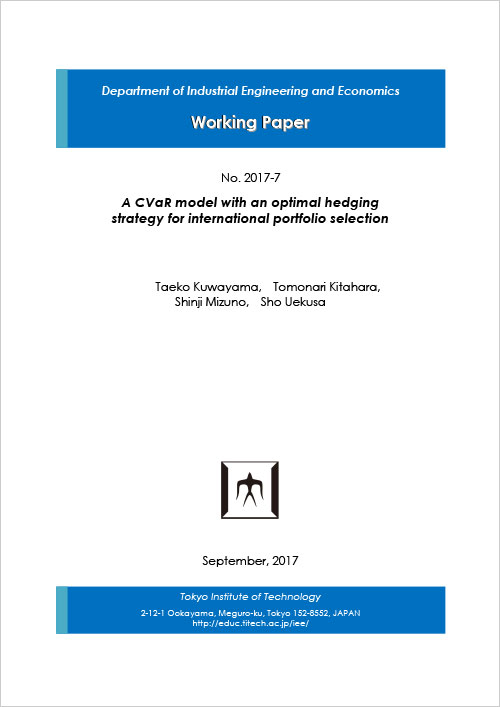# 経営工学系ワーキングペーパー 2017-7 掲載

2017.10.10A CVaR model with an optimal hedging strategy for international portfolio selection

Taeko Kuwayama, Tomonari Kitahara, Shinji Mizuno, Sho Uekusa

For international portfolio selection, currency risks affect the performance of a portfolio. We can reduce currency risks by introducing forward contracts. In this paper, we propose an optimization model for international portfolio selection with hedging strategies using forward contracts. As a risk measure, we use Conditional Value at Risk (CVaR) of the portfolio's return. The model decides both a portfolio and hedge ratios simultaneously such that CVaR is minimized under the condition that the expected return of the portfolio is not less than a predetermined value. At first, the model is formulated as a nonlinear programming problem. Then we will show that the model is equivalently converted to a linear programming problem. We conduct numerical experiments to evaluate the proposed model. As a result, we observe that the proposed model automatically adjusts the portfolio and the hedge ratios according to market environments and this adjustment leads to a stable performance of the portfolio.